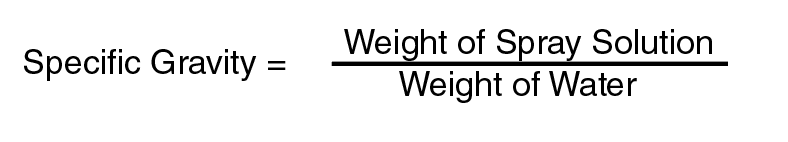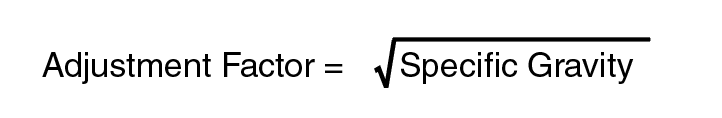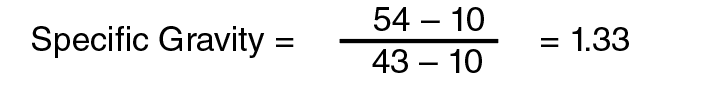# Using Water to Calibrate Sprayers for Fertilizers and Other Liquid Solutions

Sprayer calibration and nozzle testing is done using only water in the sprayer tank. For most commonly used water-based spray solutions, the addition of pesticide chemistries does very little to affect nozzle flow rates. The other commonly labeled carrier for pesticides is liquid fertilizer solutions. When using liquid fertilizer solutions as the carrier liquid for chemicals, the nozzle flow rate can vary greatly at higher flow rates. Much of this difference is due to the difference in the density of the liquid carrier. Liquid fertilizers tend to be much heavier than water per gallon. Table 1 provides some adjustment factors that allow calibration with only water when using other carrier liquids.

For example, according to the herbicide label, 28 percent UAN liquid fertilizer is to be used as a carrier for a burndown herbicide application at an application rate of 20 GPA. If calibrating using water only, at what application rate (GPA) should you calibrate the sprayer?

Using Table 1, 28% percent UAN (28-0-0) has a density of 10.65 pounds per gallon and an adjustment factor of 1.13. Multiplying the label rate by the adjustment factor will give the water-only rate used when calibrating our sprayer:

28%UAN Rate (gpa)=20 gpa×1.13=22.6 gpa

Table 1. Carrier Liquid Specific Gravity and Adjustment Factors.

Density of Solution
(lb/gal)
kerosene 6.6 0.79 0.89
Water 8.33 1 1
Salt Water 8.5 1.02 1.01
20% CaCl 9.91 1.19 1.09
28-0-0 10.65 1.28 1.13
30-0-0 10.8 1.3 1.14
32-0-0 11.06 1.33 1.15
7-21-7 11.2 1.34 1.16
10-34-0 11.4 1.37 1.17
12-0-0-26 11.5 1.38 1.17
11-37-0 11.6 1.39 1.18
12 1.68 1.3
14 1.68 1.3

The sprayer must be calibrated to apply almost 23 gallons per acre using only water, so when loaded with 28 percent UAN, the correct rate of 20 GPA will be applied. This means that at the same nozzle and pressure settings, the liquid fertilizer flows out of the nozzle at a lower rate than water.

If the spray solution is a mixture not listed in Table 1, the adjustment factor can be calculated.

Step 1. Using a glass jar with at least a 1 quart capacity (or other dedicated pesticide measuring container), fill the jar with a sample of spray solution and record the weight of the spray solution. (Any type of scale that will weigh to the closest ounce or gram will work.) Measure the same volume of water and record the weight. Be sure to subtract the weight of the measuring container before weighing each or record the empty container weight before beginning.

Step 2. Calculate the specific gravity of the spray solution using Equation (1).

(1)Step 3. Calculate the adjustment factor by taking the square root of the specific gravity

(2)For Example, a spray solution of 32 percent UAN, adjuvants and herbicides were collected in a quart jar. The total weight of the full jar was 54 ounces. When filled with water, the jar weighed 43 ounces. The empty jar on the scale was 10 ounces. What is the adjustment factor for this solution?

Using Equation (1):Using Equation (2):Any label rate (GPA) would have to be multiplied by 1.15 to determine the water-only calibration application rate (GPA) required.

The same adjustment factor can be used to determine the correct nozzle flow rate to use when calibrating with water. For example:

A boomless sprayer has been calibrated with water to apply 20 GPA, resulting in a nozzle flow rate of 2.25 gallons per minute (GPM) at 35psi. If applying at the same ground speed, the 32 percent UAN solution discussed previously, what is the new nozzle flow rate for calibration with water?

The 32 percent UAN solution was determined to have an adjustment factor of 1.15:

32%UAN Nozzle Flow Rate (GPM)=2.25 GPM×1.15=2.59 GPM

The sprayer would need to be recalibrated by increasing the pressure until the nozzle produced a 2.59 GPM flow rate using water only. This new pressure setting should produce a flow of 2.25 GPM when using the 32 percent UAN solution.

John M. Long
Extension Agricultural Engineer